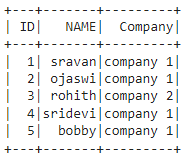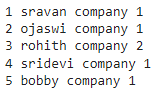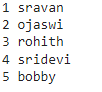GeeksforGeeks App
Open AppBrowser
Continue

# How to Iterate over rows and columns in PySpark dataframe

In this article, we will discuss how to iterate rows and columns in PySpark dataframe.

Create the dataframe for demonstration:

## Python3

 `# importing module``import` `pyspark` `# importing sparksession from pyspark.sql module``from` `pyspark.sql ``import` `SparkSession` `# creating sparksession and giving an app name``spark ``=` `SparkSession.builder.appName(``'sparkdf'``).getOrCreate()` `# list  of employee data``data ``=` `[[``"1"``, ``"sravan"``, ``"company 1"``],``        ``[``"2"``, ``"ojaswi"``, ``"company 1"``],``        ``[``"3"``, ``"rohith"``, ``"company 2"``],``        ``[``"4"``, ``"sridevi"``, ``"company 1"``],``        ``[``"5"``, ``"bobby"``, ``"company 1"``]]` `# specify column names``columns ``=` `[``'ID'``, ``'NAME'``, ``'Company'``]` `# creating a dataframe from the lists of data``dataframe ``=` `spark.createDataFrame(data, columns)` `dataframe.show()`

Output:## Method 1: Using collect()

This method will collect all the rows and columns of the dataframe and then loop through it using for loop. Here an iterator is used to iterate over a loop from the collected elements using the collect() method.

Syntax:

```for itertator in dataframe.collect():
print(itertator["column_name"],...............)```

where,

• dataframe is the input dataframe
• iterator is used to collect rows
• column_name is the column to iterate rows

Example: Here we are going to iterate all the columns in the dataframe with collect() method and inside the for loop, we are specifying iterator[‘column_name’] to get column values.

## Python3

 `# importing module``import` `pyspark` `# importing sparksession from pyspark.sql module``from` `pyspark.sql ``import` `SparkSession` `# creating sparksession and giving an app name``spark ``=` `SparkSession.builder.appName(``'sparkdf'``).getOrCreate()` `# list  of employee data``data ``=` `[[``"1"``, ``"sravan"``, ``"company 1"``],``        ``[``"2"``, ``"ojaswi"``, ``"company 1"``],``        ``[``"3"``, ``"rohith"``, ``"company 2"``],``        ``[``"4"``, ``"sridevi"``, ``"company 1"``],``        ``[``"5"``, ``"bobby"``, ``"company 1"``]]` `# specify column names``columns ``=` `[``'ID'``, ``'NAME'``, ``'Company'``]` `# creating a dataframe from the lists of data``dataframe ``=` `spark.createDataFrame(data, columns)` `# using collect``for` `i ``in` `dataframe.collect():``    ``# display``    ``print``(i[``"ID"``], i[``"NAME"``], i[``"Company"``])`

Output:## Method 2: Using toLocalIterator()

It will return the iterator that contains all rows and columns in RDD. It is similar to the collect() method, But it is in rdd format, so it is available inside the rdd method. We can use the toLocalIterator() with rdd like:

`dataframe.rdd.toLocalIterator()`

For iterating the all rows and columns we are iterating this inside an for loop

Syntax:

```for itertator in dataframe.rdd.toLocalIterator():
print(itertator["column_name"],...............)```

where,

• dataframe is the input dataframe
• iterator is used to collect rows
• column_name is the column to iterate rows

Example: Here we are going to iterate all the columns in the dataframe with toLocalIterator() method and inside the for loop, we are specifying iterator[‘column_name’] to get column values.

## Python3

 `# importing module``import` `pyspark` `# importing sparksession from pyspark.sql module``from` `pyspark.sql ``import` `SparkSession` `# creating sparksession and giving an app name``spark ``=` `SparkSession.builder.appName(``'sparkdf'``).getOrCreate()` `# list  of employee data``data ``=` `[[``"1"``, ``"sravan"``, ``"company 1"``],``        ``[``"2"``, ``"ojaswi"``, ``"company 1"``],``        ``[``"3"``, ``"rohith"``, ``"company 2"``],``        ``[``"4"``, ``"sridevi"``, ``"company 1"``],``        ``[``"5"``, ``"bobby"``, ``"company 1"``]]` `# specify column names``columns ``=` `[``'ID'``, ``'NAME'``, ``'Company'``]` `# creating a dataframe from the lists of data``dataframe ``=` `spark.createDataFrame(data, columns)` `# using toLocalIterator()``for` `i ``in` `dataframe.rdd.toLocalIterator():``  ` `    ``# display``    ``print``(i[``"ID"``], i[``"NAME"``], i[``"Company"``])`

Output:## Method 3: Using iterrows()

This will iterate rows. Before that, we have to convert our PySpark dataframe into Pandas dataframe using toPandas() method. This method is used to iterate row by row in the dataframe.

Syntax: dataframe.toPandas().iterrows()

Example: In this example, we are going to iterate three-column rows using iterrows() using for loop.

## Python3

 `# importing module``import` `pyspark` `# importing sparksession from pyspark.sql module``from` `pyspark.sql ``import` `SparkSession` `# creating sparksession and giving an app name``spark ``=` `SparkSession.builder.appName(``'sparkdf'``).getOrCreate()` `# list  of employee data``data ``=` `[[``"1"``, ``"sravan"``, ``"company 1"``],``        ``[``"2"``, ``"ojaswi"``, ``"company 1"``],``        ``[``"3"``, ``"rohith"``, ``"company 2"``],``        ``[``"4"``, ``"sridevi"``, ``"company 1"``],``        ``[``"5"``, ``"bobby"``, ``"company 1"``]]` `# specify column names``columns ``=` `[``'ID'``, ``'NAME'``, ``'Company'``]` `# creating a dataframe from the lists of data``dataframe ``=` `spark.createDataFrame(data, columns)` `# using iterrows()``for` `index, row ``in` `dataframe.toPandas().iterrows():``    ``# display with index``    ``print``(row[``0``], row[``1``], row[``2``])`

Output:## Method 4: Using select()

The select() function is used to select the number of columns. we are then using the collect() function to get the rows through for loop.

The select method will select the columns which are mentioned and get the row data using collect() method. This method will collect rows from the given columns.

Syntax: dataframe.select(“column1″,…………,”column n”).collect()

Example: Here we are going to select ID and Name columns from the given dataframe using the select() method

## Python3

 `# importing module``import` `pyspark` `# importing sparksession from pyspark.sql module``from` `pyspark.sql ``import` `SparkSession` `# creating sparksession and giving an app name``spark ``=` `SparkSession.builder.appName(``'sparkdf'``).getOrCreate()` `# list  of employee data``data ``=` `[[``"1"``, ``"sravan"``, ``"company 1"``],``        ``[``"2"``, ``"ojaswi"``, ``"company 1"``],``        ``[``"3"``, ``"rohith"``, ``"company 2"``],``        ``[``"4"``, ``"sridevi"``, ``"company 1"``],``        ``[``"5"``, ``"bobby"``, ``"company 1"``]]` `# specify column names``columns ``=` `[``'ID'``, ``'NAME'``, ``'Company'``]` `# creating a dataframe from the lists of data``dataframe ``=` `spark.createDataFrame(data, columns)` `# select only id and company``for` `rows ``in` `dataframe.select(``"ID"``, ``"Name"``).collect():``       ``# display``    ``print``(rows[``0``], rows[``1``])`

Output:## Method 5: Using list comprehension

This will act as a loop to get each row and finally we can use for loop to get particular columns, we are going to iterate the data in the given column using the collect() method through rdd.

Syntax: dataframe.rdd.collect()

Example: Here we are going to iterate rows in NAME column.

## Python3

 `# importing module``import` `pyspark` `# importing sparksession from pyspark.sql module``from` `pyspark.sql ``import` `SparkSession` `# creating sparksession and giving an app name``spark ``=` `SparkSession.builder.appName(``'sparkdf'``).getOrCreate()` `# list  of employee data``data ``=` `[[``"1"``, ``"sravan"``, ``"company 1"``],``        ``[``"2"``, ``"ojaswi"``, ``"company 1"``],``        ``[``"3"``, ``"rohith"``, ``"company 2"``],``        ``[``"4"``, ``"sridevi"``, ``"company 1"``],``        ``[``"5"``, ``"bobby"``, ``"company 1"``]]` `# specify column names``columns ``=` `[``'ID'``, ``'NAME'``, ``'Company'``]` `# creating a dataframe from the lists of data``dataframe ``=` `spark.createDataFrame(data, columns)` `# select name column``for` `i ``in` `[j[``"NAME"``] ``for` `j ``in` `dataframe.rdd.collect()]:``    ``print``(i)`

Output:

```sravan
ojaswi
rohith
sridevi
bobby```

## Method 6: Using map()

In this method, we will use map() function, which returns a new vfrom a given dataframe or RDD.  The map() function is used with the lambda function to iterate through each row of the pyspark Dataframe.

For looping through each row using map() first we have to convert the PySpark dataframe into RDD because map() is performed on RDD’s only, so first convert into RDD it then use map() in which, lambda function for iterating through each row and stores the new RDD in some variable then convert back that new RDD into Dataframe using toDF() by passing schema into it.

Syntax:

```rdd=dataframe.rdd.map(lambda loop: (
loop["column1"],...,loop["columnn"]) )
rdd.toDF(["column1",.......,"columnn"]).collect()```

Example: Here we are going to iterate ID and NAME column

## Python3

 `# importing module``import` `pyspark` `# importing sparksession from pyspark.sql module``from` `pyspark.sql ``import` `SparkSession` `# creating sparksession and giving an app name``spark ``=` `SparkSession.builder.appName(``'sparkdf'``).getOrCreate()` `# list  of employee data``data ``=` `[[``"1"``, ``"sravan"``, ``"company 1"``],``        ``[``"2"``, ``"ojaswi"``, ``"company 1"``],``        ``[``"3"``, ``"rohith"``, ``"company 2"``],``        ``[``"4"``, ``"sridevi"``, ``"company 1"``],``        ``[``"5"``, ``"bobby"``, ``"company 1"``]]` `# specify column names``columns ``=` `[``'ID'``, ``'NAME'``, ``'Company'``]` `# creating a dataframe from the lists of data``dataframe ``=` `spark.createDataFrame(data, columns)` `# select id and name column using map()``rdd ``=` `dataframe.rdd.``map``(``lambda` `loop: (``    ``loop[``"ID"``], loop[``"NAME"``]))` `# convert to dataframe and display``rdd.toDF([``"ID"``, ``"NAME"``]).collect()`

Output:

```[Row(ID='1', NAME='sravan'),
Row(ID='2', NAME='ojaswi'),
Row(ID='3', NAME='rohith'),
Row(ID='4', NAME='sridevi'),
Row(ID='5', NAME='bobby')]```

My Personal Notes arrow_drop_up